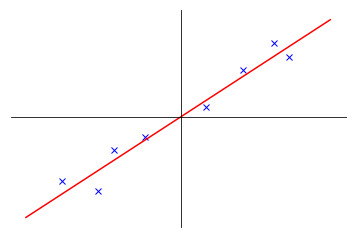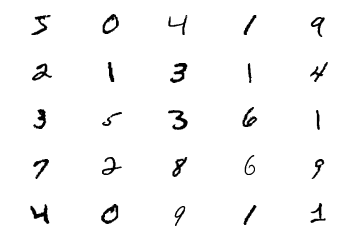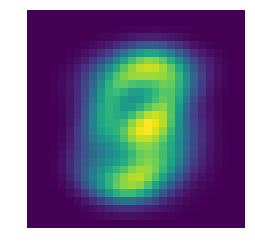This article is my notes on Principal Component Analysis (PCA) for Lecture 14 and 15 of Machine Learning by Andrew Ng. Given a set of high dimensional data $\{x^{(1)}, \dots, x^{(m)}\}$, where each $x^{(i)} \in \R^{n}$, with the assumption that these data actually roughly lie in a much smaller $k$ dimensional subspace, PCA tries to find a basis for this $k$ dimensional subspace. Let's look at a simple example:In the above figure, all blue crosses in a 2 dimensional space roughly lie on the red line, which is an 1 dimensional subspace. So we might just project all these crosses onto the 1 dimensional subspace, and treat them as 1 dimensinal data. Now the problem is how to find this subspace. Based on the figure, intuition tells us to find a direction such that the sum of distances from points to the subspace is minimal. Or equivalently, we ought to find a direction on which the sum of projections of data on the subspace is maximal. Formulate this idea into mathematics, we have to find a unit vector u to maximize

$$\frac{1}{m}\sum_{i=1}^m (u^T \cdot x^{(i)})^2.$$

Note that

$$(u^T \cdot x^{(i)})^2 = \left((x^{(i)})^T \cdot u\right)\left(u^T \cdot x^{(i)}\right) = (x^{(i)})^T \cdot (uu^T) \cdot x^{(i)},$$

so the formulation is the same as maximizing

$$\frac{1}{m}\sum_{i=1}^m (x^{(i)})^T \cdot (uu^T) \cdot x^{(i)},$$

subject to the condition that $u^T u = 1$. An easy calculation with Lagrange multiplier, we see that $u$ must be the principal eigenvector of the matrix

$$\Sigma = \frac{1}{m}\sum_{i=1}^m x^{(i)}(x^{(i)})^T.$$

We are now ready to state the algorithm for PCA, which typically consists of three steps.

Step 1: normalize data to zero mean and unit variance.

The given data usually do not lie in a vectorial subspace, but in an affine subspace. By normalizing data to zero mean, I think, we shift the data from an affine space to a vectorial subspace. For a data point, components might have different scaling. To elliminate this discrepancy, we normalize the data so that they have unit variance. More concretely, the following manipulations are applied to the original data.

(a) find the mean: $\mu = \frac{1}{m} \sum_{i=1}^m x^{(i)}$;
(b) replace $x^{(i)}$ by $x^{(i)}-\mu$;
(c) calculate the variance: $\sigma_j^2 = \frac{1}{m} \sum_{i=1}^m (x_j^{(i)})^T \cdot x^{(i)}$;
(d) replace $x_j^{(i)}$ by $\frac{x_j^{(i)}}{\sigma_j}$

We can skip the normalization if all components are of same scale.

Step 2: compute $\Sigma = \frac{1}{m} \sum_{i=1}^m x^{(i)}(x^{(i)})^T$.

This step actually compute the covariance matrix of the normalized data.

Step 3: find top $k$ eigenvectors of $\Sigma$.

These $k$ eigenvectors then span the subspace we want to find.

As an example, I am going to use the mnist dataset and calculate the principal eigenvector of the dataset.

import sys
py = 'Python ' + '.'.join(map(str, sys.version_info[:3]))
print('Jupyter notebook with kernel: {}'.format(py))

import gzip
from urllib.request import urlopen
import numpy as np
import matplotlib.pyplot as plt

Jupyter notebook with kernel: Python 3.6.4

images_url = 'http://yann.lecun.com/exdb/mnist/train-images-idx3-ubyte.gz'
images = np.array(list(images[16:]))
m = 60000
images = images.reshape((m, 784))

r = c = 5
fig, ax = plt.subplots(r, c)
for i in range(r):
for j in range(c):
k = i * c + j
img = images[k]
img = img.reshape((28, 28))
ax[i, j].imshow(img, cmap='gray_r')
ax[i, j].axis('off')
plt.show()# normalize data to zero mean and unit variance
mean = np.mean(images, axis=0)
data = images - mean
sigma = np.sqrt(np.mean(data * data, axis=0))
for i, n in enumerate(sigma):
if n == 0:
sigma[i] = 1
data = data / sigma

# compute the covariance matrix
cov = np.dot(data.T, data) / m

# compute and sort the eigenvectors
w, v = np.linalg.eig(cov)
idx = np.argsort(w)[::-1]
w = w[idx]
v = v[:, idx]

# principal vector
pv = v[:, 0]

# visualization of principal vector
img = np.real(pv * sigma) + mean
img = img.reshape((28, 28))
plt.imshow(img)
plt.axis('off')
plt.show()This example to some extend gives us visualization of all data. For instance, we can say that the handwritten digits in the dataset mostly lie in the center of the images. Besides visualization, PCA can also pick out most important features for machine learning. For the purpose of digital recognition, we can project all images onto the top 40 eigenvectors to get the top 40 important features to represent the data.

At the end, let's discuss the implementation of PCA. When data points lie in an $n$ dimensional space, the covariance matrix $\Sigma$ will have dimension $n \times n$. When $n$ is very large compared to sample size $m$, it is computational expansive to find the eigenvectors of $\Sigma$. In this case, we can implement PCA using Singular-value decomposition.

We set $X = [x^{(1)} \cdots x^{(m)}]$, an $n \times m$ matrix. Then $\Sigma = \frac{1}{m} XX^T$. Suppose $X$ has SVD decomposition as

$$X = UDV^T,$$

where

1. $U$ is an $n \times n$ matrix consisting a set of orthonormal eigenvectors of $XX^T$
2. $V$ is a $m \times m$ matrix consisting a set of orthonormal eigenvectors of $X^TX$
3. $D$ is an $n \times m$ diagonal matrix

Thus, $U$ has all eigenvectors we need for $\Sigma$.